# 6.1 Graphs of the sine and cosine functions  (Page 8/13)

 Page 8 / 13

For the equation $\text{\hspace{0.17em}}A\text{\hspace{0.17em}}\mathrm{cos}\left(Bx+C\right)+D,$ what constants affect the range of the function and how do they affect the range?

The absolute value of the constant $\text{\hspace{0.17em}}A\text{\hspace{0.17em}}$ (amplitude) increases the total range and the constant $\text{\hspace{0.17em}}D\text{\hspace{0.17em}}$ (vertical shift) shifts the graph vertically.

How does the range of a translated sine function relate to the equation $\text{\hspace{0.17em}}y=A\text{\hspace{0.17em}}\mathrm{sin}\left(Bx+C\right)+D?$

How can the unit circle be used to construct the graph of $\text{\hspace{0.17em}}f\left(t\right)=\mathrm{sin}\text{\hspace{0.17em}}t?$

At the point where the terminal side of $\text{\hspace{0.17em}}t\text{\hspace{0.17em}}$ intersects the unit circle, you can determine that the $\text{\hspace{0.17em}}\mathrm{sin}\text{\hspace{0.17em}}t\text{\hspace{0.17em}}$ equals the y -coordinate of the point.

## Graphical

For the following exercises, graph two full periods of each function and state the amplitude, period, and midline. State the maximum and minimum y -values and their corresponding x -values on one period for $\text{\hspace{0.17em}}x>0.\text{\hspace{0.17em}}$ Round answers to two decimal places if necessary.

$f\left(x\right)=2\mathrm{sin}\text{\hspace{0.17em}}x$

$f\left(x\right)=\frac{2}{3}\mathrm{cos}\text{\hspace{0.17em}}x$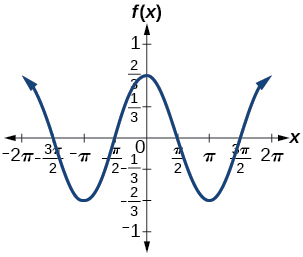amplitude: $\text{\hspace{0.17em}}\frac{2}{3};\text{\hspace{0.17em}}$ period: $\text{\hspace{0.17em}}2\pi ;\text{\hspace{0.17em}}$ midline: $\text{\hspace{0.17em}}y=0;\text{\hspace{0.17em}}$ maximum: $\text{\hspace{0.17em}}y=\frac{2}{3}\text{\hspace{0.17em}}$ occurs at $\text{\hspace{0.17em}}x=0;\text{\hspace{0.17em}}$ minimum: $\text{\hspace{0.17em}}y=-\frac{2}{3}\text{\hspace{0.17em}}$ occurs at $\text{\hspace{0.17em}}x=\pi ;\text{\hspace{0.17em}}$ for one period, the graph starts at 0 and ends at $\text{\hspace{0.17em}}2\pi$

$f\left(x\right)=-3\mathrm{sin}\text{\hspace{0.17em}}x$

$f\left(x\right)=4\mathrm{sin}\text{\hspace{0.17em}}x$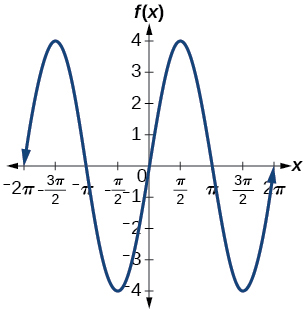amplitude: 4; period: $\text{\hspace{0.17em}}2\pi ;\text{\hspace{0.17em}}$ midline: $\text{\hspace{0.17em}}y=0;\text{\hspace{0.17em}}$ maximum $\text{\hspace{0.17em}}y=4\text{\hspace{0.17em}}$ occurs at $\text{\hspace{0.17em}}x=\frac{\pi }{2};\text{\hspace{0.17em}}$ minimum: $\text{\hspace{0.17em}}y=-4\text{\hspace{0.17em}}$ occurs at $\text{\hspace{0.17em}}x=\frac{3\pi }{2};\text{\hspace{0.17em}}$ one full period occurs from $\text{\hspace{0.17em}}x=0\text{\hspace{0.17em}}$ to $\text{\hspace{0.17em}}x=2\pi$

$f\left(x\right)=2\mathrm{cos}\text{\hspace{0.17em}}x$

$f\left(x\right)=\mathrm{cos}\left(2x\right)$amplitude: 1; period: $\text{\hspace{0.17em}}\pi ;\text{\hspace{0.17em}}$ midline: $\text{\hspace{0.17em}}y=0;\text{\hspace{0.17em}}$ maximum: $\text{\hspace{0.17em}}y=1\text{\hspace{0.17em}}$ occurs at $\text{\hspace{0.17em}}x=\pi ;\text{\hspace{0.17em}}$ minimum: $\text{\hspace{0.17em}}y=-1\text{\hspace{0.17em}}$ occurs at $\text{\hspace{0.17em}}x=\frac{\pi }{2};\text{\hspace{0.17em}}$ one full period is graphed from $\text{\hspace{0.17em}}x=0\text{\hspace{0.17em}}$ to $\text{\hspace{0.17em}}x=\pi$

$f\left(x\right)=2\text{\hspace{0.17em}}\mathrm{sin}\left(\frac{1}{2}x\right)$

$f\left(x\right)=4\text{\hspace{0.17em}}\mathrm{cos}\left(\pi x\right)$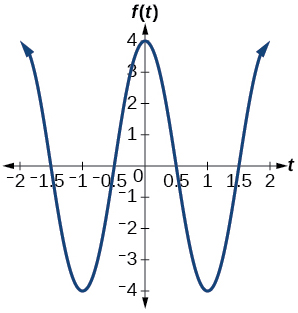amplitude: 4; period: 2; midline: $\text{\hspace{0.17em}}y=0;\text{\hspace{0.17em}}$ maximum: $\text{\hspace{0.17em}}y=4\text{\hspace{0.17em}}$ occurs at $\text{\hspace{0.17em}}x=0;\text{\hspace{0.17em}}$ minimum: $\text{\hspace{0.17em}}y=-4\text{\hspace{0.17em}}$ occurs at $\text{\hspace{0.17em}}x=1$

$f\left(x\right)=3\text{\hspace{0.17em}}\mathrm{cos}\left(\frac{6}{5}x\right)$

$y=3\text{\hspace{0.17em}}\mathrm{sin}\left(8\left(x+4\right)\right)+5$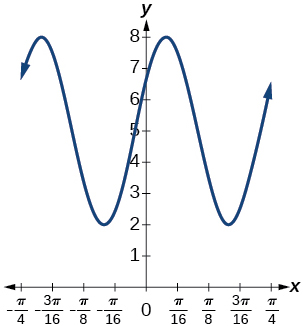amplitude: 3; period: $\text{\hspace{0.17em}}\frac{\pi }{4};\text{\hspace{0.17em}}$ midline: $\text{\hspace{0.17em}}y=5;\text{\hspace{0.17em}}$ maximum: $\text{\hspace{0.17em}}y=8\text{\hspace{0.17em}}$ occurs at $\text{\hspace{0.17em}}x=0.12;\text{\hspace{0.17em}}$ minimum: $\text{\hspace{0.17em}}y=2\text{\hspace{0.17em}}$ occurs at $\text{\hspace{0.17em}}x=0.516;\text{\hspace{0.17em}}$ horizontal shift: $\text{\hspace{0.17em}}-4;\text{\hspace{0.17em}}$ vertical translation 5; one period occurs from $\text{\hspace{0.17em}}x=0\text{\hspace{0.17em}}$ to $\text{\hspace{0.17em}}x=\frac{\pi }{4}$

$y=2\text{\hspace{0.17em}}\mathrm{sin}\left(3x-21\right)+4$

$y=5\text{\hspace{0.17em}}\mathrm{sin}\left(5x+20\right)-2$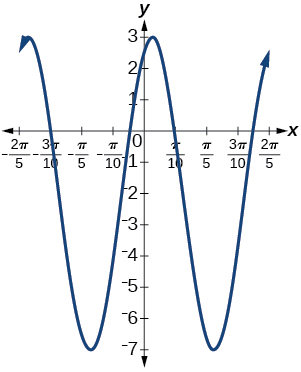amplitude: 5; period: $\text{\hspace{0.17em}}\frac{2\pi }{5};\text{\hspace{0.17em}}$ midline: $\text{\hspace{0.17em}}y=-2;\text{\hspace{0.17em}}$ maximum: $\text{\hspace{0.17em}}y=3\text{\hspace{0.17em}}$ occurs at $\text{\hspace{0.17em}}x=0.08;\text{\hspace{0.17em}}$ minimum: $\text{\hspace{0.17em}}y=-7\text{\hspace{0.17em}}$ occurs at $\text{\hspace{0.17em}}x=0.71;\text{\hspace{0.17em}}$ phase shift: $\text{\hspace{0.17em}}-4;\text{\hspace{0.17em}}$ vertical translation: $\text{\hspace{0.17em}}-2;\text{\hspace{0.17em}}$ one full period can be graphed on $\text{\hspace{0.17em}}x=0\text{\hspace{0.17em}}$ to $\text{\hspace{0.17em}}x=\frac{2\pi }{5}$

For the following exercises, graph one full period of each function, starting at $\text{\hspace{0.17em}}x=0.\text{\hspace{0.17em}}$ For each function, state the amplitude, period, and midline. State the maximum and minimum y -values and their corresponding x -values on one period for $\text{\hspace{0.17em}}x>0.\text{\hspace{0.17em}}$ State the phase shift and vertical translation, if applicable. Round answers to two decimal places if necessary.

$f\left(t\right)=2\mathrm{sin}\left(t-\frac{5\pi }{6}\right)$

$f\left(t\right)=-\mathrm{cos}\left(t+\frac{\pi }{3}\right)+1$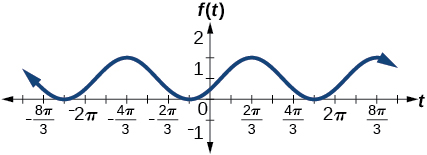amplitude: 1 ; period: $\text{\hspace{0.17em}}2\pi ;\text{\hspace{0.17em}}$ midline: $\text{\hspace{0.17em}}y=1;\text{\hspace{0.17em}}$ maximum: $\text{\hspace{0.17em}}y=2\text{\hspace{0.17em}}$ occurs at $\text{\hspace{0.17em}}x=2.09;\text{\hspace{0.17em}}$ maximum: $\text{\hspace{0.17em}}y=2\text{\hspace{0.17em}}$ occurs at $\text{\hspace{0.17em}}t=2.09;\text{\hspace{0.17em}}$ minimum: $\text{\hspace{0.17em}}y=0\text{\hspace{0.17em}}$ occurs at $\text{\hspace{0.17em}}t=5.24;\text{\hspace{0.17em}}$ phase shift: $\text{\hspace{0.17em}}-\frac{\pi }{3};\text{\hspace{0.17em}}$ vertical translation: 1; one full period is from $\text{\hspace{0.17em}}t=0\text{\hspace{0.17em}}$ to $\text{\hspace{0.17em}}t=2\pi$

$f\left(t\right)=4\mathrm{cos}\left(2\left(t+\frac{\pi }{4}\right)\right)-3$

$f\left(t\right)=-\mathrm{sin}\left(\frac{1}{2}t+\frac{5\pi }{3}\right)$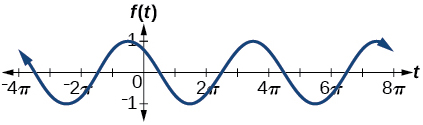amplitude: 1; period: $\text{\hspace{0.17em}}4\pi ;\text{\hspace{0.17em}}$ midline: $\text{\hspace{0.17em}}y=0;\text{\hspace{0.17em}}$ maximum: $\text{\hspace{0.17em}}y=1\text{\hspace{0.17em}}$ occurs at $\text{\hspace{0.17em}}t=11.52;\text{\hspace{0.17em}}$ minimum: $\text{\hspace{0.17em}}y=-1\text{\hspace{0.17em}}$ occurs at $\text{\hspace{0.17em}}t=5.24;\text{\hspace{0.17em}}$ phase shift: $\text{\hspace{0.17em}}-\frac{10\pi }{3};\text{\hspace{0.17em}}$ vertical shift: 0

$f\left(x\right)=4\mathrm{sin}\left(\frac{\pi }{2}\left(x-3\right)\right)+7$

Determine the amplitude, midline, period, and an equation involving the sine function for the graph shown in [link] .

amplitude: 2; midline: $\text{\hspace{0.17em}}y=-3;\text{\hspace{0.17em}}$ period: 4; equation: $\text{\hspace{0.17em}}f\left(x\right)=2\mathrm{sin}\left(\frac{\pi }{2}x\right)-3$

Determine the amplitude, period, midline, and an equation involving cosine for the graph shown in [link] .

Determine the amplitude, period, midline, and an equation involving cosine for the graph shown in [link] .

amplitude: 2; period: 5; midline: $\text{\hspace{0.17em}}y=3;\text{\hspace{0.17em}}$ equation: $\text{\hspace{0.17em}}f\left(x\right)=-2\mathrm{cos}\left(\frac{2\pi }{5}x\right)+3$

Determine the amplitude, period, midline, and an equation involving sine for the graph shown in [link] .

Determine the amplitude, period, midline, and an equation involving cosine for the graph shown in [link] .

amplitude: 4; period: 2; midline: $\text{\hspace{0.17em}}y=0;\text{\hspace{0.17em}}$ equation: $\text{\hspace{0.17em}}f\left(x\right)=-4\mathrm{cos}\left(\pi \left(x-\frac{\pi }{2}\right)\right)$

Determine the amplitude, period, midline, and an equation involving sine for the graph shown in [link] .

Determine the amplitude, period, midline, and an equation involving cosine for the graph shown in [link] .

amplitude: 2; period: 2; midline $\text{\hspace{0.17em}}y=1;\text{\hspace{0.17em}}$ equation: $\text{\hspace{0.17em}}f\left(x\right)=2\mathrm{cos}\left(\pi x\right)+1$

Determine the amplitude, period, midline, and an equation involving sine for the graph shown in [link] .

## Algebraic

For the following exercises, let $\text{\hspace{0.17em}}f\left(x\right)=\mathrm{sin}\text{\hspace{0.17em}}x.$

On $\text{\hspace{0.17em}}\left[0,2\pi \right),$ solve $\text{\hspace{0.17em}}f\left(x\right)=0.$

On $\text{\hspace{0.17em}}\left[0,2\pi \right),$ solve $\text{\hspace{0.17em}}f\left(x\right)=\frac{1}{2}.$

$\frac{\pi }{6},\frac{5\pi }{6}$

Evaluate $\text{\hspace{0.17em}}f\left(\frac{\pi }{2}\right).$

On $\text{\hspace{0.17em}}\left[0,2\pi \right),f\left(x\right)=\frac{\sqrt{2}}{2}.\text{\hspace{0.17em}}$ Find all values of $\text{\hspace{0.17em}}x.$

$\frac{\pi }{4},\frac{3\pi }{4}$

On $\text{\hspace{0.17em}}\left[0,2\pi \right),$ the maximum value(s) of the function occur(s) at what x -value(s)?

On $\text{\hspace{0.17em}}\left[0,2\pi \right),$ the minimum value(s) of the function occur(s) at what x -value(s)?

$\frac{3\pi }{2}$

Show that $\text{\hspace{0.17em}}f\left(-x\right)=-f\left(x\right).\text{\hspace{0.17em}}$ This means that $\text{\hspace{0.17em}}f\left(x\right)=\mathrm{sin}\text{\hspace{0.17em}}x\text{\hspace{0.17em}}$ is an odd function and possesses symmetry with respect to ________________.

For the following exercises, let $\text{\hspace{0.17em}}f\left(x\right)=\mathrm{cos}\text{\hspace{0.17em}}x.$

On $\text{\hspace{0.17em}}\left[0,2\pi \right),$ solve the equation $\text{\hspace{0.17em}}f\left(x\right)=\mathrm{cos}\text{\hspace{0.17em}}x=0.$

$\frac{\pi }{2},\frac{3\pi }{2}$

On $\text{\hspace{0.17em}}\left[0,2\pi \right),$ solve $\text{\hspace{0.17em}}f\left(x\right)=\frac{1}{2}.$

On $\text{\hspace{0.17em}}\left[0,2\pi \right),$ find the x -intercepts of $\text{\hspace{0.17em}}f\left(x\right)=\mathrm{cos}\text{\hspace{0.17em}}x.$

$\frac{\pi }{2},\frac{3\pi }{2}$

On $\text{\hspace{0.17em}}\left[0,2\pi \right),$ find the x -values at which the function has a maximum or minimum value.

On $\text{\hspace{0.17em}}\left[0,2\pi \right),$ solve the equation $\text{\hspace{0.17em}}f\left(x\right)=\frac{\sqrt{3}}{2}.$

$\frac{\pi }{6},\frac{11\pi }{6}$

## Technology

Graph $\text{\hspace{0.17em}}h\left(x\right)=x+\mathrm{sin}\text{\hspace{0.17em}}x\text{\hspace{0.17em}}$ on $\text{\hspace{0.17em}}\left[0,2\pi \right].\text{\hspace{0.17em}}$ Explain why the graph appears as it does.

Graph $\text{\hspace{0.17em}}h\left(x\right)=x+\mathrm{sin}\text{\hspace{0.17em}}x\text{\hspace{0.17em}}$ on $\text{\hspace{0.17em}}\left[-100,100\right].\text{\hspace{0.17em}}$ Did the graph appear as predicted in the previous exercise?

The graph appears linear. The linear functions dominate the shape of the graph for large values of $\text{\hspace{0.17em}}x.$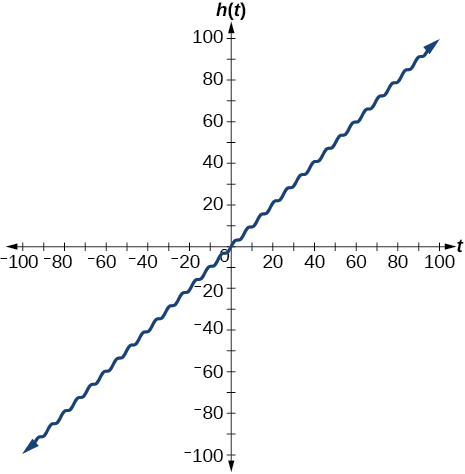Graph $\text{\hspace{0.17em}}f\left(x\right)=x\text{\hspace{0.17em}}\mathrm{sin}\text{\hspace{0.17em}}x\text{\hspace{0.17em}}$ on $\text{\hspace{0.17em}}\left[0,2\pi \right]\text{\hspace{0.17em}}$ and verbalize how the graph varies from the graph of $\text{\hspace{0.17em}}f\left(x\right)=\mathrm{sin}\text{\hspace{0.17em}}x.$

Graph $\text{\hspace{0.17em}}f\left(x\right)=x\text{\hspace{0.17em}}\mathrm{sin}\text{\hspace{0.17em}}x\text{\hspace{0.17em}}$ on the window $\text{\hspace{0.17em}}\left[-10,10\right]\text{\hspace{0.17em}}$ and explain what the graph shows.

The graph is symmetric with respect to the y -axis and there is no amplitude because the function is not periodic.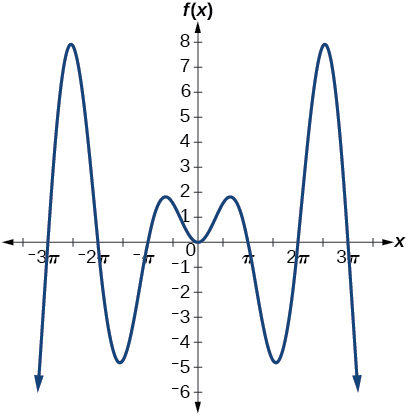Graph $\text{\hspace{0.17em}}f\left(x\right)=\frac{\mathrm{sin}\text{\hspace{0.17em}}x}{x}\text{\hspace{0.17em}}$ on the window $\text{\hspace{0.17em}}\left[-5\pi ,5\pi \right]\text{\hspace{0.17em}}$ and explain what the graph shows.

## Real-world applications

A Ferris wheel is 25 meters in diameter and boarded from a platform that is 1 meter above the ground. The six o’clock position on the Ferris wheel is level with the loading platform. The wheel completes 1 full revolution in 10 minutes. The function $\text{\hspace{0.17em}}h\left(t\right)\text{\hspace{0.17em}}$ gives a person’s height in meters above the ground t minutes after the wheel begins to turn.

1. Find the amplitude, midline, and period of $\text{\hspace{0.17em}}h\left(t\right).$
2. Find a formula for the height function $\text{\hspace{0.17em}}h\left(t\right).$
3. How high off the ground is a person after 5 minutes?
1. Amplitude: 12.5; period: 10; midline: $\text{\hspace{0.17em}}y=13.5;$
2. $h\left(t\right)=12.5\mathrm{sin}\left(\frac{\pi }{5}\left(t-2.5\right)\right)+13.5;$
3. 26 ft

a colony of bacteria is growing exponentially doubling in size every 100 minutes. how much minutes will it take for the colony of bacteria to triple in size
I got 300 minutes. is it right?
Patience
no. should be about 150 minutes.
Jason
It should be 158.5 minutes.
Mr
ok, thanks
Patience
what is the importance knowing the graph of circular functions?
can get some help basic precalculus
What do you need help with?
Andrew
how to convert general to standard form with not perfect trinomial
can get some help inverse function
ismail
Rectangle coordinate
how to find for x
it depends on the equation
Robert
whats a domain
The domain of a function is the set of all input on which the function is defined. For example all real numbers are the Domain of any Polynomial function.
Spiro
foci (–7,–17) and (–7,17), the absolute value of the differenceof the distances of any point from the foci is 24.
difference between calculus and pre calculus?
give me an example of a problem so that I can practice answering
x³+y³+z³=42
Robert
dont forget the cube in each variable ;)
Robert
of she solves that, well ... then she has a lot of computational force under her command ....
Walter
what is a function?
I want to learn about the law of exponent
explain this
what is functions?
A mathematical relation such that every input has only one out.
Spiro
yes..it is a relationo of orders pairs of sets one or more input that leads to a exactly one output.
Mubita
Is a rule that assigns to each element X in a set A exactly one element, called F(x), in a set B.
RichieRich
If the plane intersects the cone (either above or below) horizontally, what figure will be created?

#### Get Jobilize Job Search Mobile App in your pocket Now!ByByBy Edgar DelgadoBy Rohini AjayBy OpenStaxBy Cath YuBy Abishek DevarajBy Hannah ShethBy Anonymous UserByBy Bonnie HurstBy OpenStax